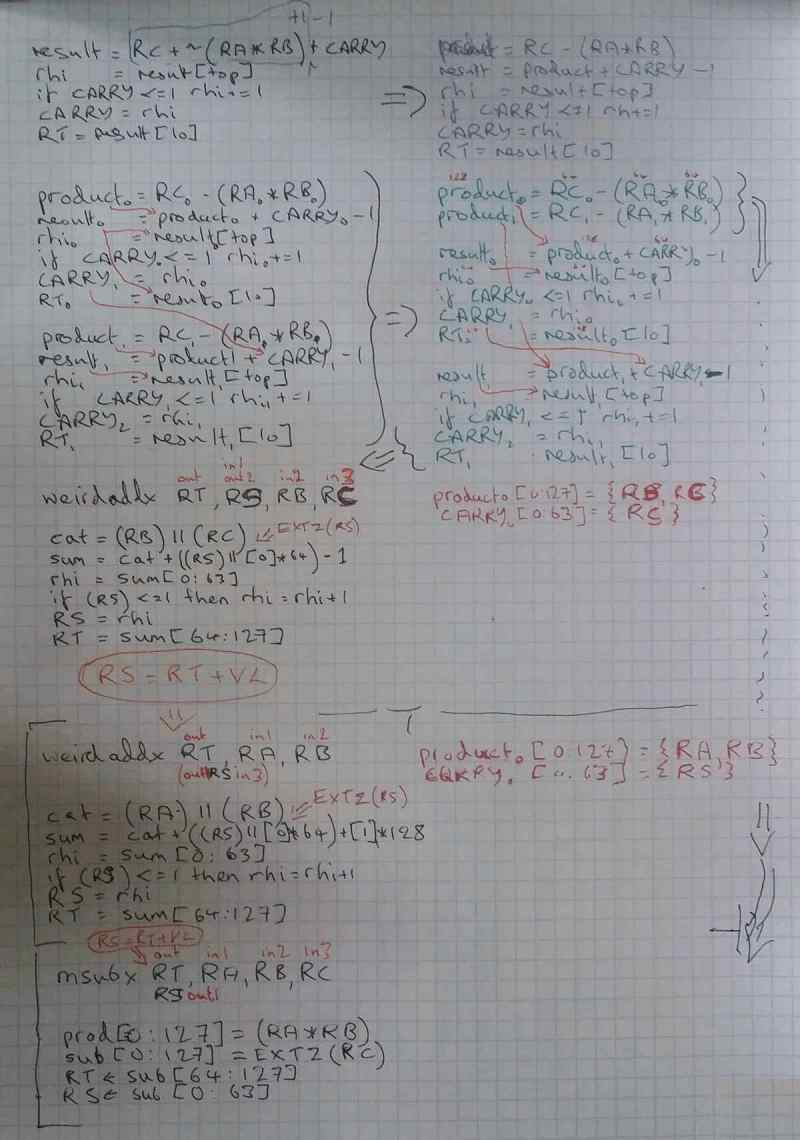# big integer multiply

## Variant 1

Row-based multiply using temporary vector. Simple implementation of Knuth M: https://git.libre-soc.org/?p=libreriscv.git;a=blob;f=openpower/sv/bitmanip/mulmnu.c;hb=HEAD

``````      for (i = 0; i < m; i++) {
unsigned product = u[i]*v[j] + w[i + j];
phi[i] = product>>16;
plo[i] = product;
}
for (i = 0; i < m; i++) {
t = (phi[i]<<16) | plo[i] + k;
w[i + j] = t;          // (I.e., t & 0xFFFF).
k = t >> 16;
}
``````

maddx RT, RA, RB, RC (RS=RT+VL for SVP64, RS=RT+1 for scalar)

``````prod[0:127] = (RA) * (RB)
sum[0:127] = EXTZ(RC) + prod
RT <- sum[64:127]
RS <- sum[0:63]
``````

addxd RT, RA, RB (RS=RB+VL for SVP64, RS=RB+1 for scalar)

``````cat[0:127] = (RS) || (RB)
sum[0:127] = cat + EXTZ(RA)
RA = sum[0:63]
RT = sum[64:127]
``````

These two combine as, simply:

``````# assume VL=8, therefore RS starts at r8.v
# q           : r16
# multiplier  : r17
# multiplicand: r20.v
# carry       : r18
li r18, 0
# here, RS=RB+VL, therefore again RS starts at r8.v
``````

## Variant 2

``````      for (i = 0; i < m; i++) {
unsigned product = u[i]*v[j] + k;
k = product>>16;
plo[i] = product; // & 0xffff
}
k = 0;
for (i = 0; i < m; i++) {
t = plo[i] + w[i + j] + k;
w[i + j] = t;          // (I.e., t & 0xFFFF).
k = t >> 16; // carry: should only be 1 bit
}
``````

``````prod[0:127] = (RA) * (RB)
sum[0:127] = EXTZ(RC) + prod
RT <- sum[64:127]
RC <- sum[0:63]
``````

# big integer division

the most efficient division algorithm is probably Knuth's Algorithm D (with modifications from the exercises section of his book) which is O(n2) and uses 2N-by-N-bit div/rem

an oversimplified version of the knuth algorithm d with 32-bit words is: (TODO find original: https://raw.githubusercontent.com/hcs0/Hackers-Delight/master/divmnu64.c.txt

``````void div(uint32_t *n, uint32_t *d, uint32_t* q, int n_bytes, int d_bytes) {
// assumes d != 0, also n, d, and q have their most-significant-word in index 0
int q_bytes = n_bytes - d_bytes;
for(int i = 0; i < q_bytes / sizeof(n); i++) {
// calculate guess for quotient word
q[i] = (((uint64_t)n[i] << 32) + n[i + 1]) / d;
// n -= q[i] * d
uint32_t carry = 0, carry2 = 0;
for(int j = d_bytes / sizeof(d) - 1; j >= 0; j--) {
uint64_t v = (uint64_t)q[i] * d[j] + carry;
carry = v >> 32;
v = (uint32_t)v;
v = n[i + j] - v + carry2;
carry2 = v >> 32; // either ~0 or 0
n[i + j] = v;
}
// fixup if carry2 != 0
}
// now remainder is in n and quotient is in q
}
``````

The key loop may be implemented with a 4-in, 2-out mul-twin-add (which is too much):

``````On Sat, Apr 16, 2022, 22:06 Jacob Lifshay <programmerjake@gmail.com> wrote:
and a mrsubcarry (the one actually needed by bigint division):

# for big_c - big_a * word_b
result <- RC + ~(RA * RB) + CARRY # wrong, needs further thought
CARRY <- HIGH_HALF(result)
RT <- LOW_HALF(result)

turns out, after some checking with 4-bit words, afaict the correct
algorithm for mrsubcarry is:

# for big_c - big_a * word_b
result <- RC + ~(RA * RB) + CARRY
result_high <- HIGH_HALF(result)
if CARRY <= 1 then # unsigned comparison
result_high <- result_high + 1
end
CARRY <- result_high
RT <- LOW_HALF(result)

afaict, that'll make the following algorithm work:

so the inner loop in the bigint division algorithm would end up being
(assuming n, d, and q all fit in registers):

li r3, 1 # carry in for subtraction
mtspr CARRY, r3 # init carry spr
setvl loop_count
sv.mrsubcarry rn.v, rd.v, rq.s, rn.v
``````

This algorithm may be morphed into a pair of Vector operations by temporary storage of the products.

``````      uint32_t borrow = 0;
for(int i = 0; i <= n; i++) {
uint32_t vn_i = i < n ? vn[i] : 0;
uint64_t value = un[i + j] - (uint64_t)qhat * vn_i;
plo[i] = value & 0xffffffffLL;
phi[i] = value >> 32;
}
for(int i = 0; i <= n; i++) {
uint64_t value = (((uint64_t)phi[i]<<32) | plo[i]) - borrow;
borrow = ~(value >> 32)+1; // -(uint32_t)(value >> 32);
un[i + j] = (uint32_t)value;
}
bool need_fixup = borrow != 0;
``````

Transformation of 4-in, 2-out into a pair of operations:

• 3-in, 2-out `msubx RT, RA, RB, RC` producing {RT,RS} where RS=RT+VL
• 3-in, 2-out `subxd RT, RA, RB` a hidden RS=RT+VL as input, RA dualA trick used in the DCT and FFT twin-butterfly instructions, originally borrowed from `lq` and LD/ST-with-update, is to have a second hidden (implicit) destination register, RS. RS is calculated as RT+VL, where all scalar operations assume VL=1. With `sv.msubx` creating a pair of Vector results, `sv.weirdaddx` correspondingly has to pick the pairs up, containing the split lo-hi 128-bit products, in order to carry on the algorithm.

msubx RT, RA, RB, RC (RS=RT+VL for SVP64, RS=RT+1 for scalar)

``````prod[0:127] = (RA) * (RB)
sub[0:127] = EXTZ(RC) - prod
RT <- sub[64:127]
RS <- sub[0:63]
``````

subxd RT, RA, RB (RS=RB+VL for SVP64, RS=RB+1 for scalar)

``````cat[0:127] = (RS) || (RB)
sum[0:127] = cat - EXTS(RA)
RA = ~sum[0:63] + 1
RT = sum[64:127]
``````

These two combine as, simply:

``````# assume VL=8, therefore RS starts at r8.v
# q       : r16
# dividend: r17
# divisor : r20.v
# carry   : r18
li r18, 0
sv.msubx r0.v, r16, r17, r20.v
# here, RS=RB+VL, therefore again RS starts at r8.v
sv.subxd r0.v, r18, r0.v
``````

As a result, a big-integer subtract and multiply may be carried out in only 3 instructions, one of which is setting a scalar integer to zero.

An Rc=1 variant tests not against RT but RA, which allows detection of a fixup in Knuth Algorithm D: the condition where RA is not zero.